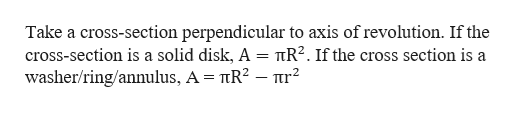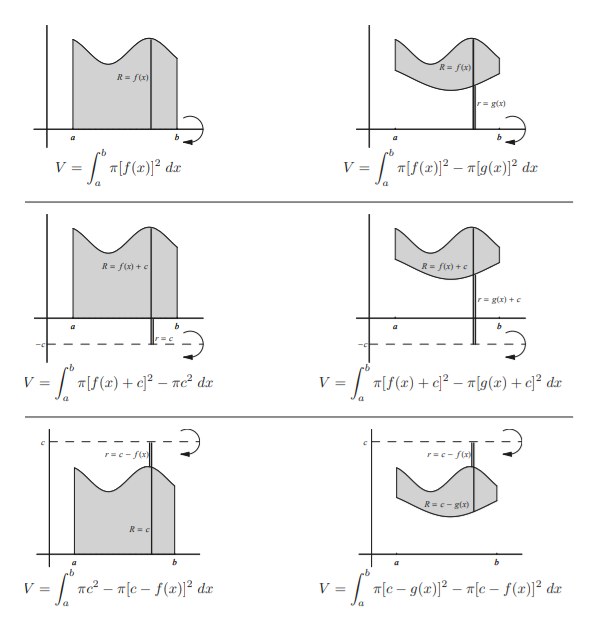# For the solids of revolution, how do I know when to add or subtract from the axis of revolution such as when y=3, y=-2, or x=-2, x=2, for both disk, washer, and cylindrical shell method, when finding the volume?

Question
67 views

For the solids of revolution, how do I know when to add or subtract from the axis of revolution such as when y=3, y=-2, or x=-2, x=2, for both disk, washer, and cylindrical shell method, when finding the volume?

check_circle

Step 1

For the solids of revolution, find the volume using disk, washer and cylindrical shell method.

Step 2

a) Disk or Washer Method.help_outlineImage TranscriptioncloseTake a cross-section perpendicular to axis of revolution. If the cross-section is a solid disk, A = TR2. If the cross section is a washer/ring/annulus, A = īR2 - nr fullscreen
Step 3

When the axis of revolution is horizont...help_outlineImage TranscriptioncloseR= f(x) R fx) rgx) = |[5(x)]2 dax Tf()2g()]2 dx| V = a R = fix)+ R= f(x) + e = f(2) + c]2 - ne? dz )- 9(2) + c]2 dz | rc-fx rc-fix) R =c- gix) b b b e-g()[c- f(z)]2 dz| Te2-Tcf()2 da fullscreen

### Want to see the full answer?

See Solution

#### Want to see this answer and more?

Solutions are written by subject experts who are available 24/7. Questions are typically answered within 1 hour.*

See Solution
*Response times may vary by subject and question.
Tagged in

### Calculus